Question

Strength Of Materials

5.6 For each of the following cases, determine an appropriate characteristic length Le and the corresponding Biot number Bithat is associated with the transient thermal response of the solid object. State whether the lumped capacitance approximation is valid. If temperature information is not provided, evaluate properties at T= 300 K.

(b) A long, hot AISI 304 stainless steel bar of rectangular cross section has dimensions w = 3 mm, W = 5 mm, and L = 100 mm.The bar is subjected to a coolant that provides a heat transfer coefficient of h = 15 W/m2 . K at all exposed surfaces.

(c) A long extruded aluminum (Alloy 2024) tube of inner and outer dimensions w = 20 mm and W = 24 mm, respectively, is suddenly submerged in water, resulting in a convection

(a) A toroidal shape of diameter D = 50 mm and cross-sectional area Ac = 5 mm² is of thermal conductivity k = 2.3 W/m K. The surface of the torus is exposed to a coolant corresponding to a convection coefficient of h = 50 W/m2 K.

coefficient of h = 37 W/m2 . K at the four exterior tube surfaces.The tube is plugged at both ends, trapping stagnant air inside the tube.

(d) An L = 300-mm-long solid stainless steel rod of diameter D= 13 mm and mass m = 0.328 kg is exposed to a convection coefficient of h = 30 W/m2 . K.

(e) A solid sphere of diameter D = 13 mm and thermal conductivity k = 130 W/m · Kis suspended in a large vacuum oven with internal wall temperatures of Tsur = 18°C. The initial sphere temperature is T; = 100°C, and its emissivity is e = 0.75.

(f) A long cylindrical rod of diameter D = 20 mm, density p =2300 kg/m3, specific heat c, = 1750 J/kg · K, and thermal Cp conductivity k = 16 W/m · K is suddenly exposed to convective conditions with T = 20°C. The rod is initially at a uniform temperature of T; = 200°C and reaches a spatially averaged temperature of T = 100°C at t = 225 s.

(g) Repeat part (f) but now consider a rod diameter of D = 200mm.Verified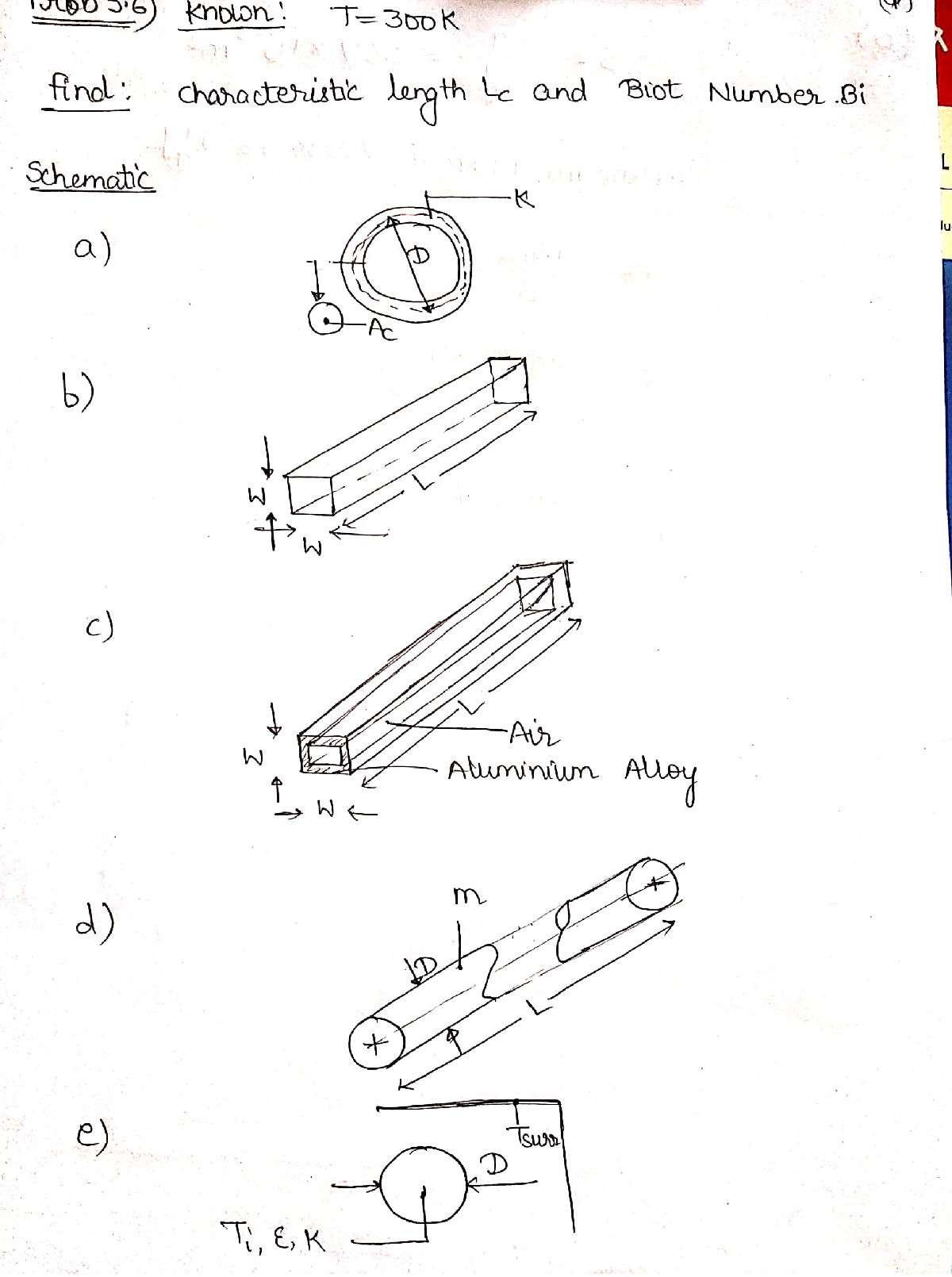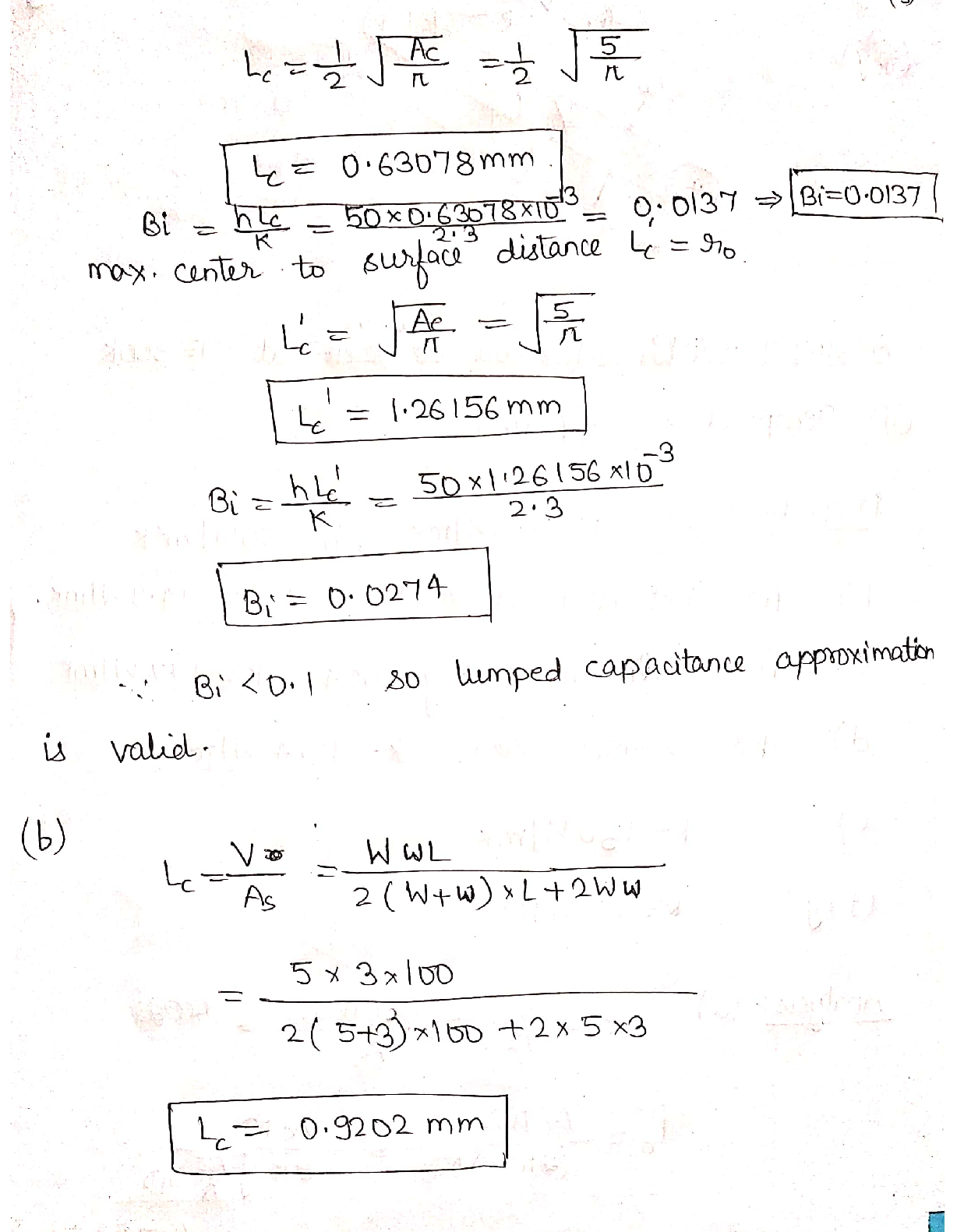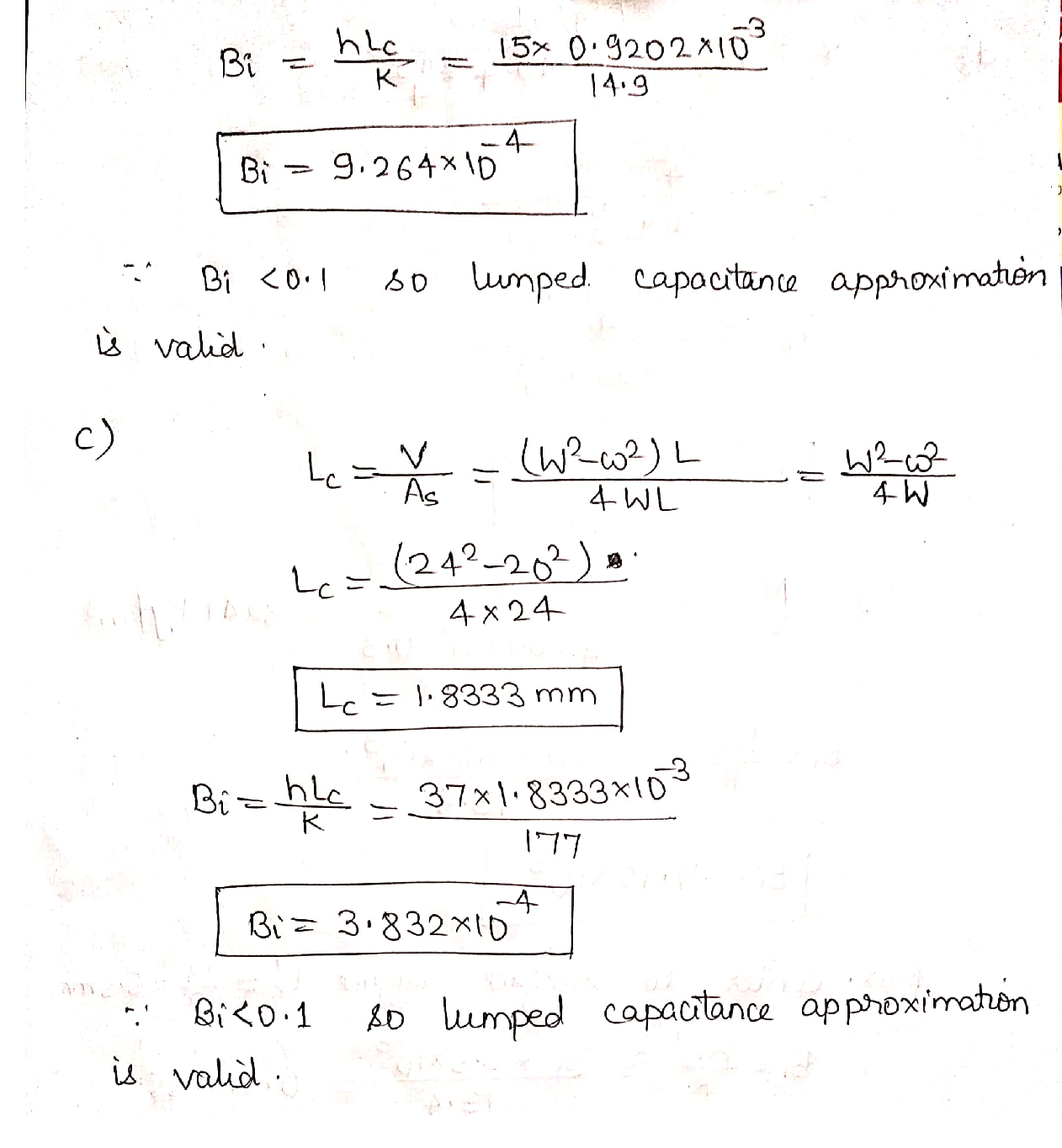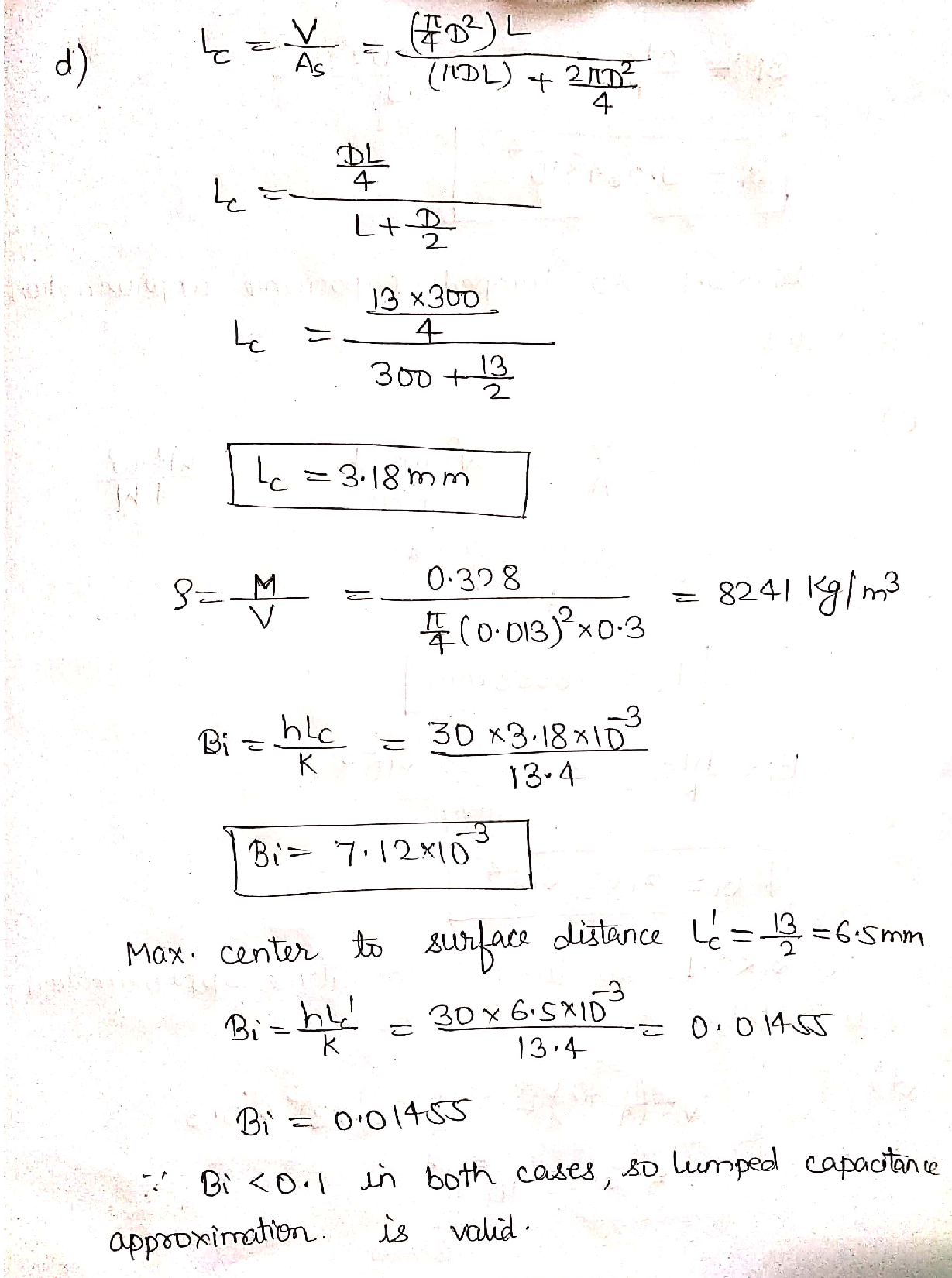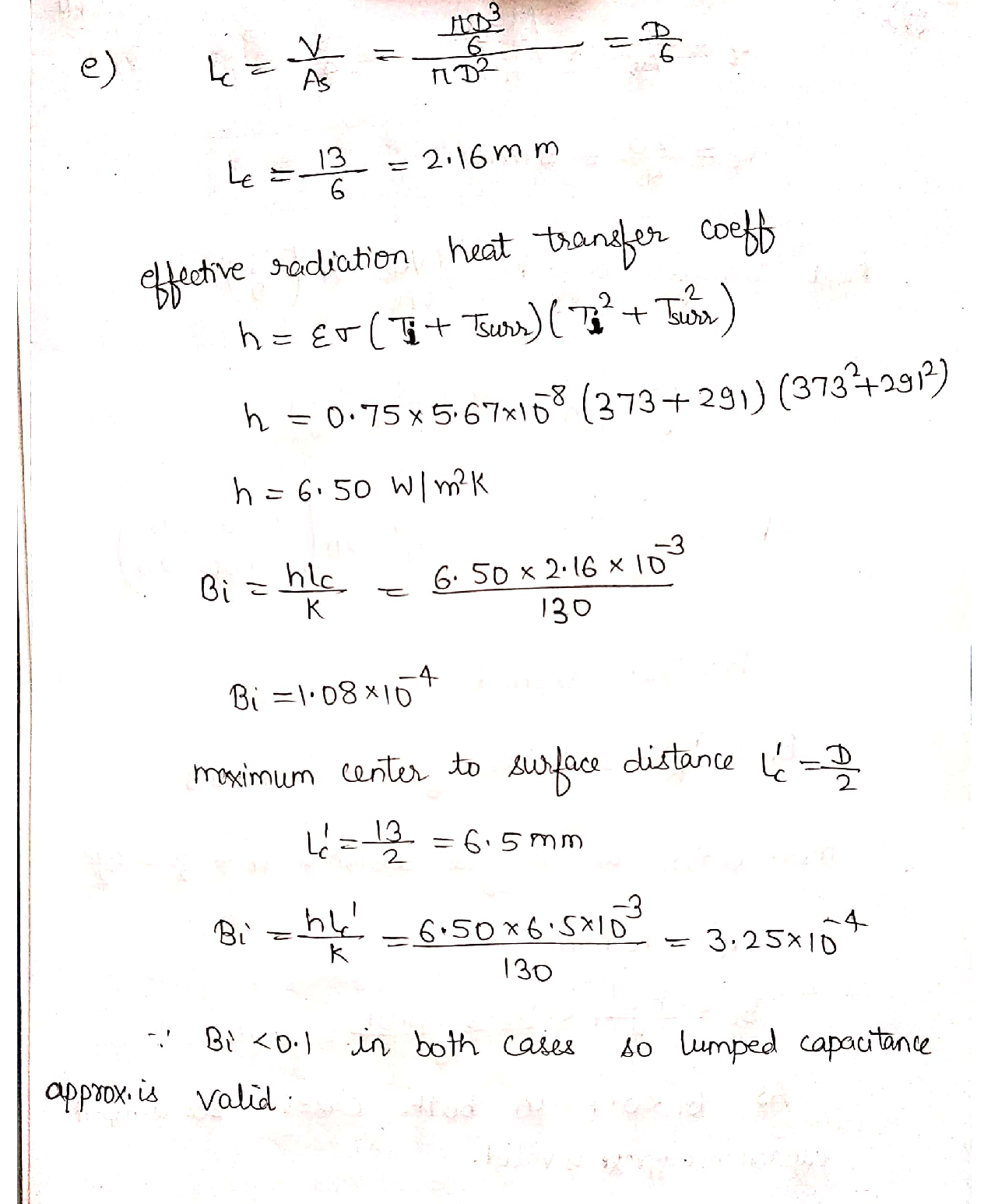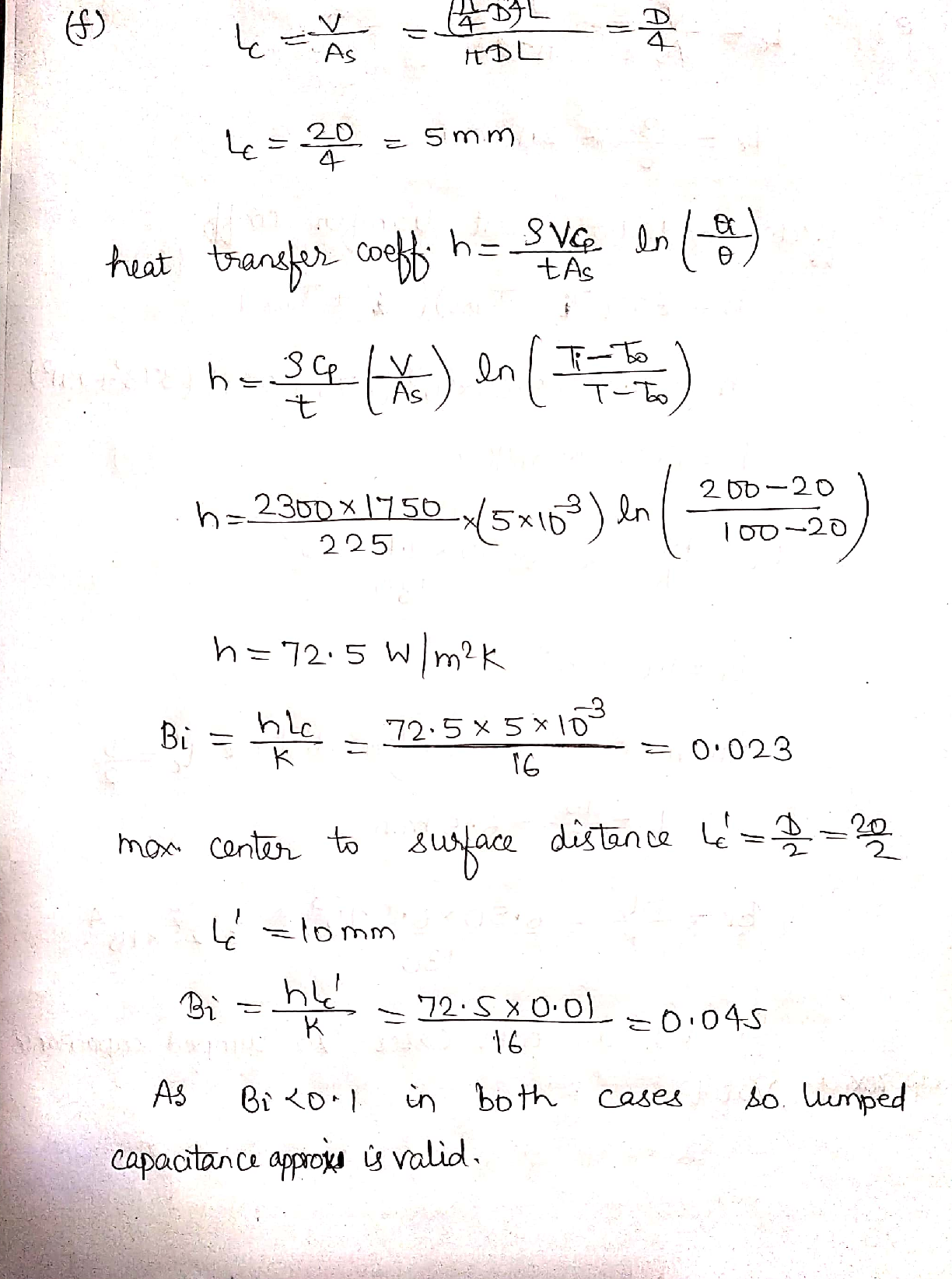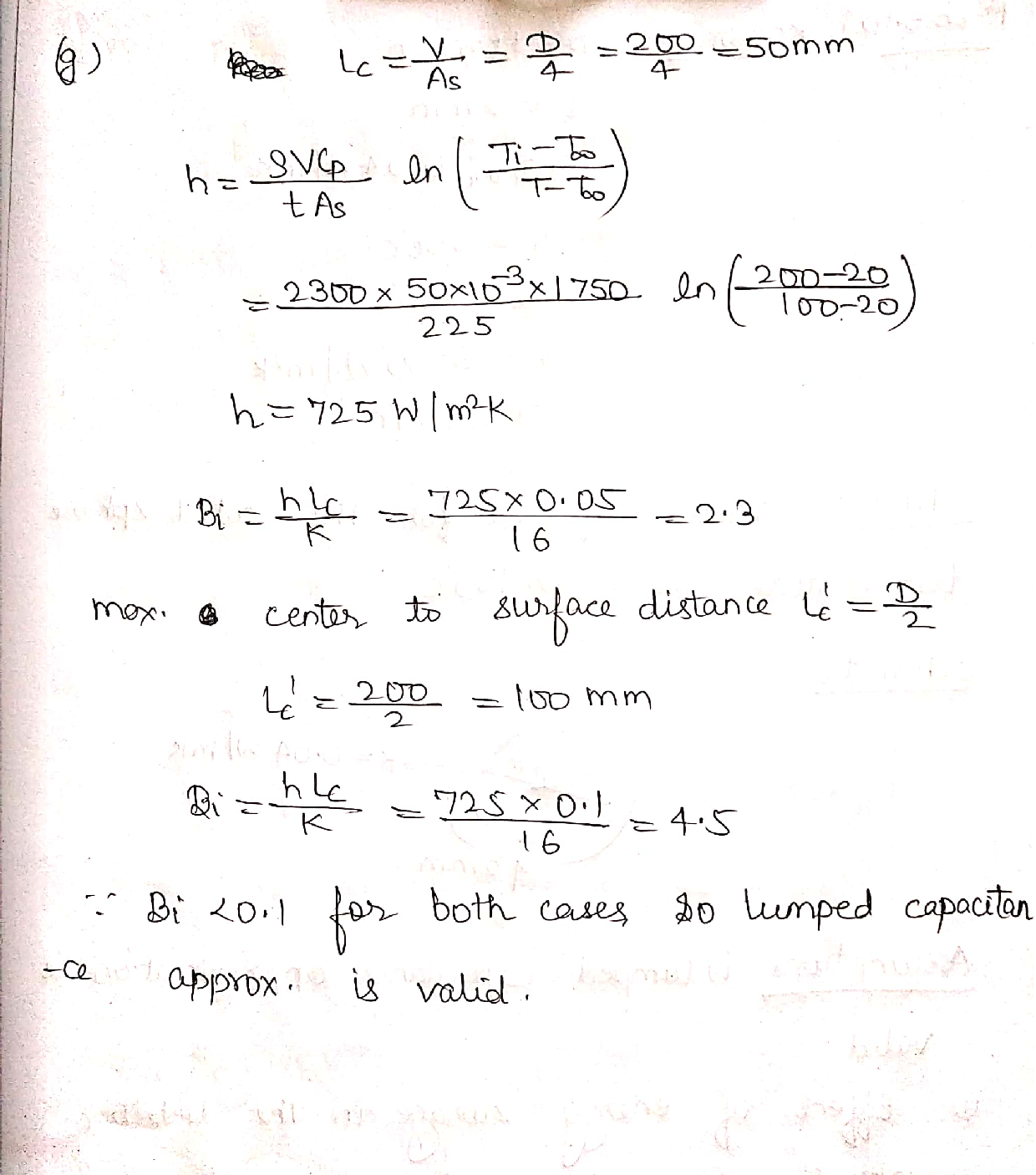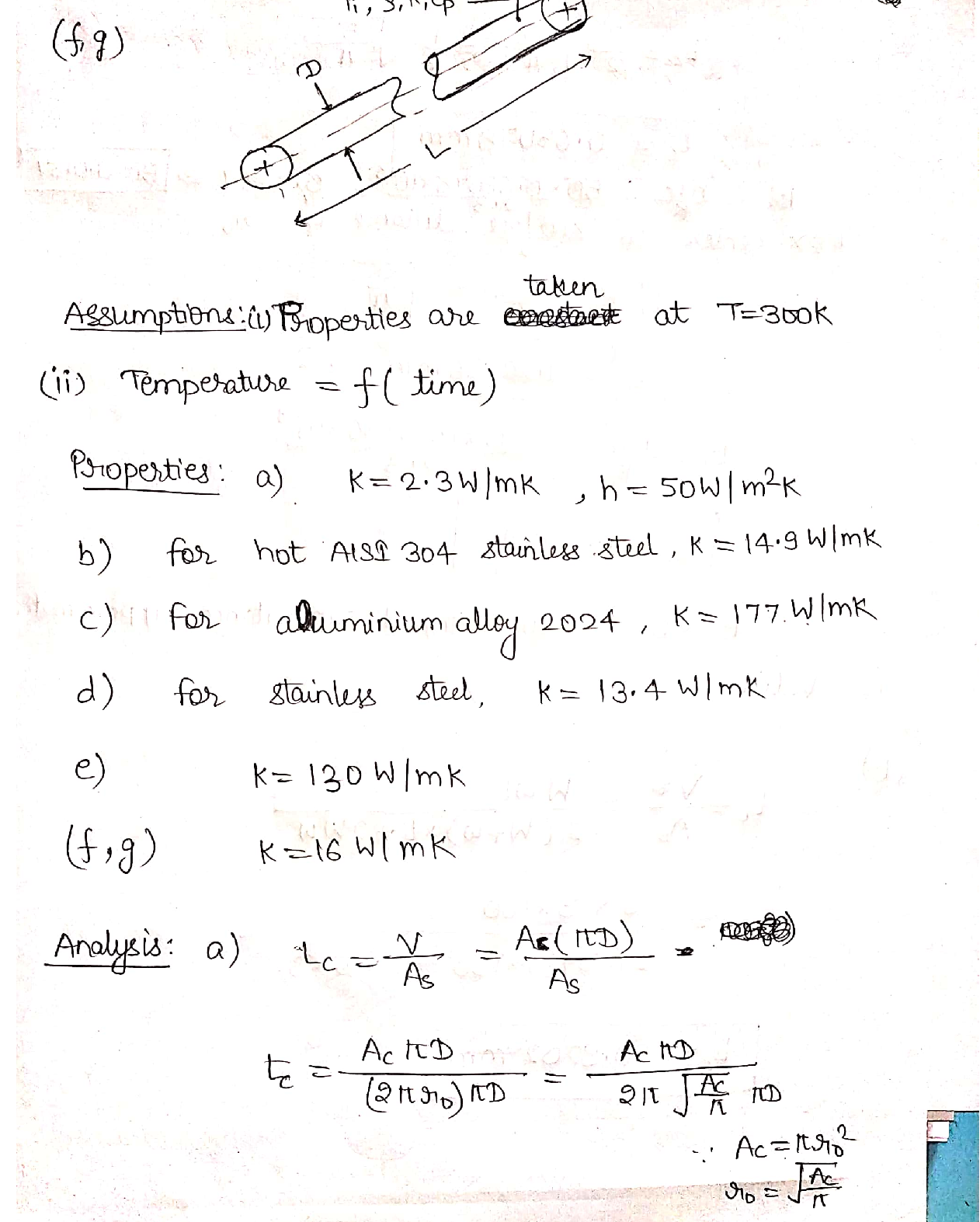### Question 45244Strength Of Materials

7-6 Bevel gears transmit torsional, bending, and axial loads to rotating shafts, such as shown in figure13-36. For this problem, the free body diagram for a shaft ABC is shown in the figure, where points Aand C are at the bearings. Line BG is on the bevel gear extended to the shaft center from point G, the contact point with the mating gear. Transmitted from the mating gear are tangential, radial, and axial forces F, = 5000 Ibf, F = 58lbf, and F, = 173 Ibf, respectively. The bearing at C supports the axial thrust load. For the shaft material, Su = 90 kpsi, Sy = 65 kpsi, and has a fully corrected endurance limit of 40kpsi. Let (Kr)a = 2.3, (K)b = 2.0 and (K), t = 1.5 where the subscripts a, b, and t represent axial, bending,and torsion, respectively. The diameter of the shaft ABC is 1.5 inches.
Determine the Design Factor (n:) and then determine the design factor ni without axial loading. How much does axial forces contribute to the design factors?

### Question 45243Strength Of Materials

7-3 The rotating steel shaft is simply supported by bearings at points B and C and is driven by a gear (not shown) which meshes with the spur gear at D, which has a 150mm pitch diameter. The force F from the drive gear acts at a pressure angle of 20°. The shaft transmits a torque to point A of TA = 340 N*m. The shaft is machined from steel with Sy = 420 MPa and Sut = 560 MPa. Using a safety factor of 2.5determine the minimum allowable diameter of the 250mm section of the shaft based on a) a static yield analysis using the distortion energy theory and b) a fatigue-failure analysis using Goodman. Assume sharp fillet radii at the bearing shoulders for estimating stress concentration factors.

### Question 45242Strength Of Materials

7-1 a) A shaft is loaded in bending and torsion such that M, = 70 N*m, T, = 45 N*m, Mm = 55 N*m, andTm = 35 N*m. For the shaft, Su = 700 MPA, and Sy = 560 MPA, and a fully corrected endurance limit of Se= 210 MPA is assumed. Let K = 2.2 and Kg = 1.8. With a design factor of 2.0 determine the minimum acceptable diameter of the shaft using the DE Goodman criteria.

### Question 44563Strength Of Materials

You have been employed as a Quality Engineer in a company involved in a high-volume production of nails for DIY. After receiving feedback from customers regarding the variation of the length of the nails, it has been decided to run some statistical analysis in order to study the quality and the distribution of the process output. For this purpose the length of a random sample of 100 nails has been measured and organised in Table Q3.
Check the data for outliers and eliminate them if any.
b) Using Chi2 criterion test if the data follows a normal distribution.
Plot a histogram and using data acquired in (b) add empirical and theoretical curves for a visual comparison.

### Question 44544Strength Of Materials

The torques shown are exerted on pulleys A, B, and C. The diameter of shaft AB is 1.3 in. and that of BC is 1.8 in. Knowing that both shafts are solid, determine the maximum shearing stressin (a) shaft AB, (b) shaft BC.

### Question 44543Strength Of Materials

Determine the torque T that causes a maximum shearing stress of 70 MPa in the steel cylindrical shaft shown.

### Question 44436Strength Of Materials

The aluminum rod AB (G = 27 GPa) is bonded to the brass rod BD (G = 39 GPa). Knowing that portion CD of the brass rod is hollow and has an inner diameter of 40 mm, determine the angle of twist at A.

### Question 44435Strength Of Materials

(a) Determine the angle of twist caused by a 40-kip -in.torque T in the 3-in.-diameter solid aluminum shaftshown (G = 3.7 × 10°psi).
(b) Solve part (a), assuming that the solid shaft has been replaced by a hollow shaft of the same outer diameter and a 1-in. inner diameter.

### Question 44305Strength Of Materials

Knowing that a force P of magnitude 750N is applied to the pedal shown, determine (a) the diameter of the pin at C for which the average shearing stress in the pin is 40 MPa, (b) the corresponding bearing stress in the pedal at C, (c) the corresponding bearing stress in each support bracket at C.

### Question 44304Strength Of Materials

Each of the four vertical links has an 8 × 36-mm uniform rectangular cross section, and each of the four pins has a 16-mm diameter. Determine the maximum value of the average normal stress in the links connecting (a) points B and D, (b) points C and E.

### Submit query